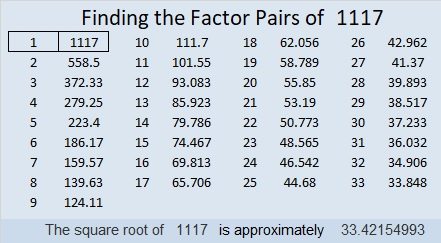# 1117 and Level 5

Can you solve this Level 5 puzzle or will it make you feel like toast? Seriously, I think it’s a little easier than the previous puzzle, so give it a try. You might just become the toast of the town!Print the puzzles or type the solution in this excel file: 12 factors 1111-1119

Now I’ll share some information about the number 1117:

• 1117 is a prime number.
• Prime factorization: 1117 is prime.
• The exponent of prime number 1117 is 1. Adding 1 to that exponent we get (1 + 1) = 2. Therefore 1117 has exactly 2 factors.
• Factors of 1117: 1, 1117
• Factor pairs: 1117 = 1 × 1117
• 1117 has no square factors that allow its square root to be simplified. √1117 ≈ 33.42155

How do we know that 1117 is a prime number? If 1117 were not a prime number, then it would be divisible by at least one prime number less than or equal to √1117 ≈ 33.4. Since 1117 cannot be divided evenly by 2, 3, 5, 7, 11, 13, 17, 19, 23, 29 or 31, we know that 1117 is a prime number.26² + 21² = 1117

1117 is the hypotenuse of a Pythagorean triple:
235-1092-1117 calculated from 26² – 21², 2(26)(21), 26² + 21²

Here’s another way we know that 1117 is a prime number: Since its last two digits divided by 4 leave a remainder of 1, and 26² + 21² = 1117 with 26 and 21 having no common prime factors, 1117 will be prime unless it is divisible by a prime number Pythagorean triple hypotenuse less than or equal to √1117 ≈ 33.4. Since 1117 is not divisible by 5, 13, 17, or 29, we know that 1117 is a prime number.

1117 is palindrome 151 in BASE 31 because 1(31²) + 5(31) + 1(1) = 1117

This site uses Akismet to reduce spam. Learn how your comment data is processed.